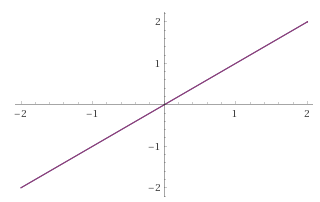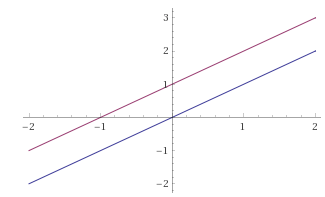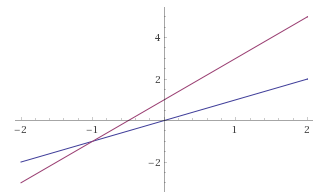# Understanding a Linear System's Three Outcomes

In two dimensions, the solution structure of a system of two equations in two unknowns can be understood in a straightforward way via pictures, with the two equations representing lines (this is why it's called linear algebra) in the $$x$$-$$y$$ (or $$x1$$ - $$x2$$) plane. A solution is any point $$(x,y)$$ ($$(x1, x2)$$) where the two lines intersect.

Which of the following three graphs is that of a linear system of two equations with two unknowns that has no solutions?